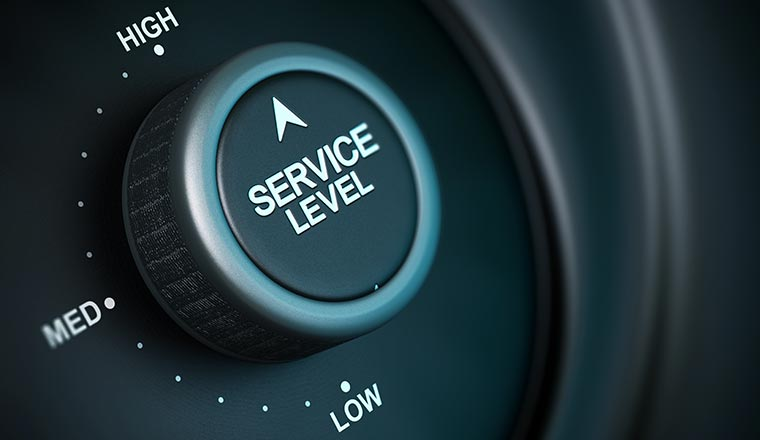# Service Level Threshold Calculations﻿
2,183
Filed under - Forum,

## Service Level Threshold Calculations

I am using the below mentioned formula to calculate the Service Level. Please let me know if this is correct –

Calls Answered within Threshold / (calls offered – Abandon within Threshold)

## Service Level

• Total calls answered = 1000
• Total calls abandoned = 60
• Total call answered within 20 seconds = 860
• Total calls abandoned within 20 seconds = 20
• Total calls abandoned after 20 seconds = 40
• Total calls abandoned within 5 seconds = 10

Formula #1:

Service Level = {(Total calls answered within threshold +calls abandoned within threshold)/(total calls answered + total calls abandoned)} * 100%

Example: (860+20)/(1000+60)*100% = 83%

Formula #2

Service level = (Total calls answered within threshold) / (Total calls answered)*100%

This formula ignores calls that abandon. Clearly this is NOT an accurate reflection of what is going on in the centre and is not one I would recommend, nor would any other people I work with.

Example: ((860)/1000))*100% = 86%

Formula #3

Service Level = (Total calls answered within threshold) / (Total calls answered + Total calls abandoned)*100%

Here you are given “credit” for all the calls you answered within your service level threshold and all calls that abandon will affect service level negatively, regardless of when they abandon.

Example: ((860)/(1000+60)) *100%= 81%

Formula #4

Service Level = (Total calls answered within threshold) / (Total calls answered + Total calls abandoned after threshold)*100%

Here calls that abandon after the threshold affect service level negatively. Call that abandon before the threshold do not affect service level.

Example: ((860)/(1000+40))*100% = 83%

Formula #5

Service Level = {(Total calls answered within threshold +calls abandoned within a shorter amount of time than the threshold)/(total calls answered + total calls abandoned)} * 100%

This formula uses a threshold to account for “short calls.” For example, if your threshold is 20 seconds, here you would say that calls that abandon before that time will count positively towards service level.

Example: ((860+10)/(1000+60))*100%= 82%

Hope the above information will help.

With thanks to Srikanth

## Contact Centre Service Level.

We have recently written a comprehensive article on the best ways to calculate Contact Centre Service Level.

With thanks to Jonty

## Calls Vs Chats Abandoned: Different Calculation

I understand the concepts/ideas behind how a contact centre would decide how they use the abandoned calls within threshold.

How about Chats? Should Chats abandoned should simply we removed from calculation? We see a lot of ghost chats… even if the speed of answer is rapid.

With thanks to luc

Author: Jonty Pearce